Covariant and Lie Derivatives

Notation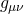is the metric, and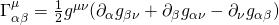are the Christoffel symbols.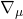is the covariant derivative, andis the partial derivative with respect to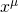.is a scalar,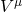is a contravariant vector, and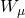is a covariant vector.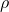is a scalar density of weight 1, and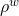is a scalar density of weight w. (Note that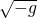is a density of weight 1, where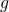is the determinant of the metric. It follows that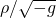is a scalar.)

Covariant Derivatives

Covariant derivatives are defined by

(1)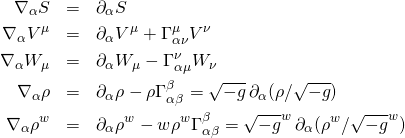The extension to tensors of different types is straightforward. Note the useful result for the divergence of a vector field: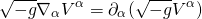Lie Derivatives

Lie derivatives with respect to a vector field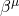are defined by

(2)The extension to tensors of different type is straightforward. Lie derivatives do not rely on the presence of a metric for their definitions. When a metric is present, they can be written in terms of covariant derivatives by simply replacing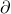with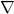in the formulas above. Note the useful result for the Lie derivative of the metric tensor:A Killing vector fieldsatisfies.